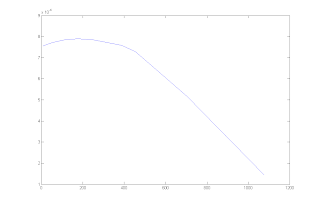## Monday, February 8, 2010

### Initial Kernel Estimation Plots

• Kernel-based methods are most popular for non-parametric estimators
• They can discover structural features in data which parametric approaches may not reveal
• Kernel performance is measured by AMISE (asymptotic mean integrated squared error)
• Below graphs were produced via Epanechnikov kernel, since Epanechnikov kernel minimizes AMISE
• For below graphs we compute a probability density estimate of a sample vector and then plot the evaluation of this probability density estimate
• Plots (lines) below correspond to probability density function for k=16 from Cocomo81 and Cocomo81 itself respectively. The stars are the sorted effort values of related datasets.